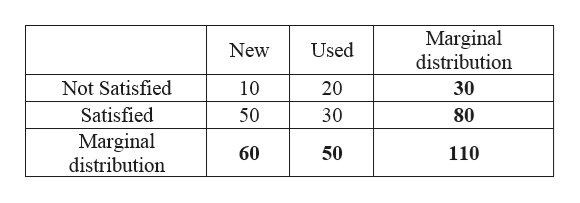# Are you more likely to be satisfied with your automobile purchase when it is new or used?  The following data represent the level of satisfaction of the buyer for both new and used cars. NewUsedNot satisfied1020Satisfied5030A) Identify the explanatory variable/response variable/row variable/column variable.B)Construct a frequency marginal distribution. NewUsedMarginal DistributionNot Satisfied1020 Satisfied5030 Marginal Distribution   C) Construct a relative frequency marginal distribution  NewUsedRelative Frequency Marginal DistributionNot Satisfied1020 Satisfied5030 Relative frequency distribution

Question

Are you more likely to be satisfied with your automobile purchase when it is new or used?  The following data represent the level of satisfaction of the buyer for both new and used cars.

 New Used Not satisfied 10 20 Satisfied 50 30

A) Identify the explanatory variable/response variable/row variable/column variable.

B)Construct a frequency marginal distribution.

 New Used Marginal Distribution Not Satisfied 10 20 Satisfied 50 30 Marginal Distribution

C) Construct a relative frequency marginal distribution

 New Used Relative Frequency Marginal Distribution Not Satisfied 10 20 Satisfied 50 30 Relative frequency distribution

check_circleExpert Solution
Step 1

The given data represent the level of satisfaction of the buyer for both new and used cars.

Step 2
1. A)

Explanatory variable:

The variable that is controlled in the experiment is called explanatory variable. Here, the explanatory variable is new or used car.

Response variable:

The response variable is the outcome variable on which comparisons of different explanatory variable will be made. Whether or not satisfied with automobile purchase when it is used or not is the outcome variable here. Therefore, the response variable is level of satisfaction of the buyer.

The row variable here is level of satisfaction of the buyer.

And, the column variable is new or used car.

Step 3

B)

Frequency marginal distribution:

The sum of row entries is the row frequency marginal distribution whereas the sum of column frequency is the c...help_outlineImage TranscriptioncloseMarginal distribution Used New Not Satisfied 10 20 30 Satisfied 50 30 80 Marginal distribution 50 60 110 fullscreen

### Want to see the full answer?

See Solution

#### Want to see this answer and more?

Solutions are written by subject experts who are available 24/7. Questions are typically answered within 1 hour*

See Solution
*Response times may vary by subject and question
Tagged in

### Other